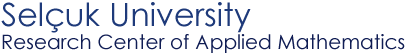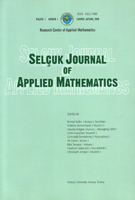Selçuk Journal of Applied Mathematics www.selcuk.edu.trSelçuk Journal of
Applied Mathematics

Summer-Autumn, 2000
Volume  1
Number 1

Research Center of
Applied Mathematics

 SJAM Summer-Autumn 2000, Volume 1 - Number 1 Algorithm with guaranteed accuracy for computing a solution to an initial value problem for linear difference equations Vladimir Vaskevich1* ,  Haydar Bulgak2, Cengiz Çinar2 1 Sobolev Institute of Mathematics, SB RAS, Novosibirsk, Russia    email: vask@math.nsc.ru  2 Research Centre of Applied Mathematics, Selçuk University, Konya, Turkey     email hbulgak@selcuk.edu.tr Received: August 31, 2000 To Prof. Dr. Chr. Zenger 60-th birthday Summary Consider an initial value problem for  simultaneous linear difference equations x(n+1) = Ax(n) + f(n), x(0) = a,    with  A  the   N´N   rational matrix,    {f(n)}    a sequence of N-dimensional rational vectors, and a a rational N-dimensional vector. The problem has a unique solution; but to compute {x(n)} in the interval [0,M] with M a nonnegative integer, we approximate the reals and carry out the elementary arithmetic operations in special way. By means of this algorithm, we solve the initial value problem for a discrete asymptotically stable matrix A with guaranteed accuracy. Key words initial value problems, simultaneous linear difference equations, algorithms with guaranteed accuracy, discrete asymptotically stable matrices Mathematics Subject Classification (1991): 65F30, 65G10 * The research was financially supported by the Scientific and Technical Research Council of Turkey (TUBITAK) in the framework of a NATO-PC Advanced Fellowships Programme. Article in PS format (103 kb) Article in ZIP format (41 kb)
 The article is available to read in  Ghostview format
 [Home] [contact]